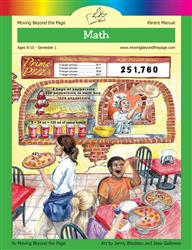# Common Core Alignment

CCSS.Math.Content.4.MD.5 - Recognize angles as geometric shapes that are formed wherever two rays share a common endpoint, and understand concepts of angle measurement:

## 5: MathUnit 3: Geometry
Lesson 11: Problem Solving
Lesson 12: Unit Test
Lesson 3: Angle Basics
Lesson 4: Measuring Angles
Lesson 5: Using a Protractor
Lesson 6: Playing with Angles